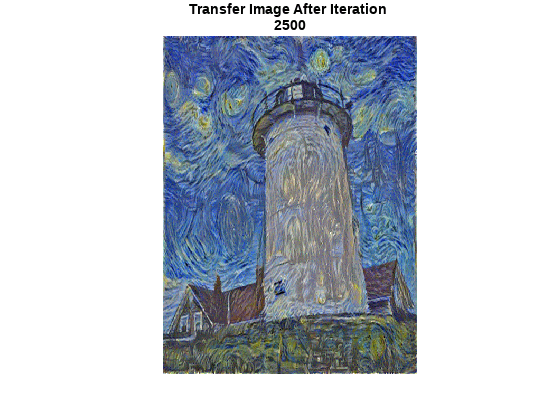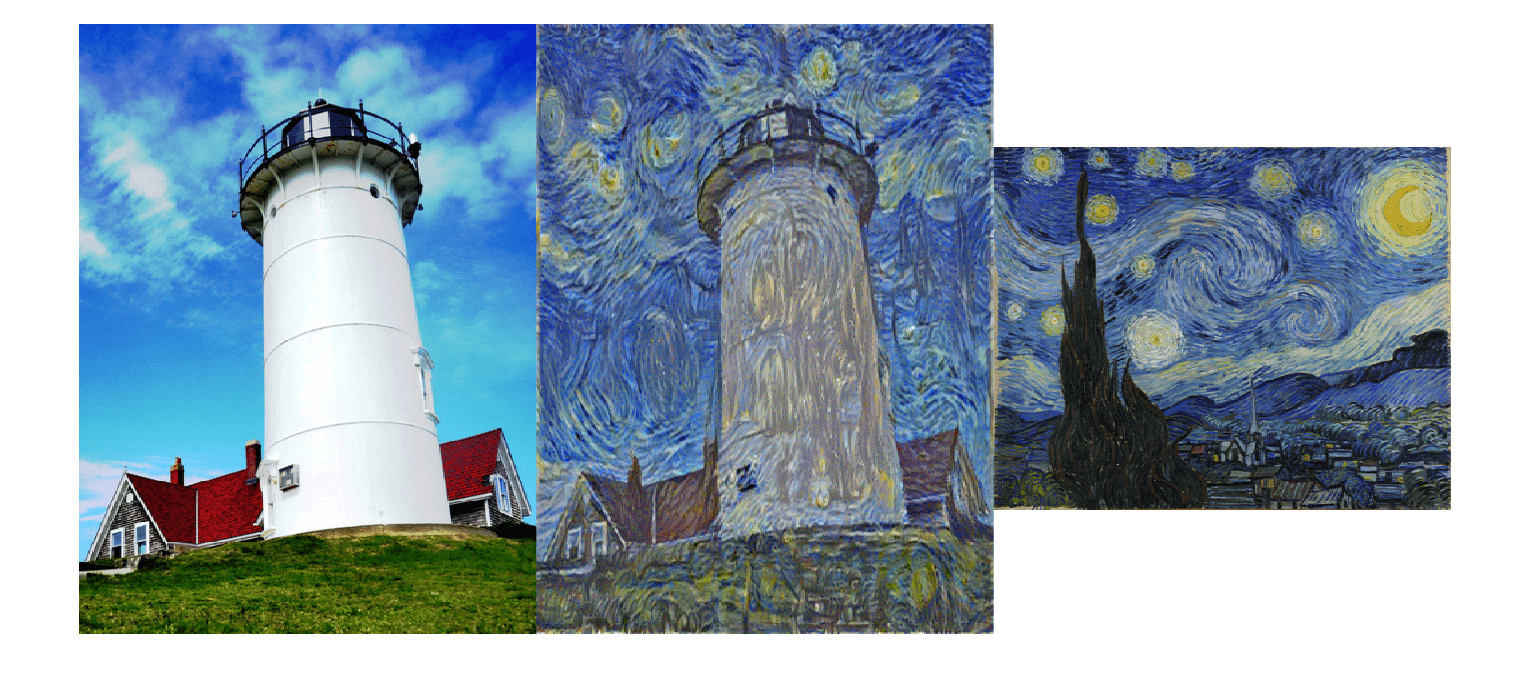# Neural Style Transfer Using Deep Learning

This example shows how to apply the stylistic appearance of one image to the scene content of a second image using a pretrained VGG-19 network.

Load the style image and content image. This example uses the distinctive Van Gogh painting "Starry Night" as the style image and a photograph of a lighthouse as the content image.

```styleImage = im2double(imread("starryNight.jpg")); contentImage = imread("lighthouse.png");```

Display the style image and content image as a montage.

`imshow(imtile({styleImage,contentImage},BackgroundColor="w"));`### Load Feature Extraction Network

In this example, you use a modified pretrained VGG-19 deep neural network to extract the features of the content and style image at various layers. These multilayer features are used to calculate respective content and style losses. The network generates the stylized transfer image using the combined loss.

To get a pretrained VGG-19 network, install `vgg19` (Deep Learning Toolbox). If you do not have the required support packages installed, then the software provides a download link.

`net = vgg19;`

To make the VGG-19 network suitable for feature extraction, remove all of the fully connected layers from the network.

```lastFeatureLayerIdx = 38; layers = net.Layers; layers = layers(1:lastFeatureLayerIdx);```

The max pooling layers of the VGG-19 network cause a fading effect. To decrease the fading effect and increase the gradient flow, replace all max pooling layers with average pooling layers .

```for l = 1:lastFeatureLayerIdx layer = layers(l); if isa(layer,"nnet.cnn.layer.MaxPooling2DLayer") layers(l) = averagePooling2dLayer(layer.PoolSize,Stride=layer.Stride,Name=layer.Name); end end```

Create a layer graph with the modified layers.

`lgraph = layerGraph(layers);`

Visualize the feature extraction network in a plot.

```plot(lgraph) title("Feature Extraction Network")```To train the network with a custom training loop and enable automatic differentiation, convert the layer graph to a `dlnetwork` object.

`dlnet = dlnetwork(lgraph);`

### Preprocess Data

Resize the style image and content image to a smaller size for faster processing.

```imageSize = [384,512]; styleImg = imresize(styleImage,imageSize); contentImg = imresize(contentImage,imageSize);```

The pretrained VGG-19 network performs classification on a channel-wise mean subtracted image. Get the channel-wise mean from the image input layer, which is the first layer in the network.

```imgInputLayer = lgraph.Layers(1); meanVggNet = imgInputLayer.Mean(1,1,:);```

The values of the channel-wise mean are appropriate for images of floating point data type with pixel values in the range [0, 255]. Convert the style image and content image to data type `single` with range [0, 255]. Then, subtract the channel-wise mean from the style image and content image.

```styleImg = rescale(single(styleImg),0,255) - meanVggNet; contentImg = rescale(single(contentImg),0,255) - meanVggNet;```

### Initialize Transfer Image

The transfer image is the output image as a result of style transfer. You can initialize the transfer image with a style image, content image, or any random image. Initialization with a style image or content image biases the style transfer process and produces a transfer image more similar to the input image. In contrast, initialization with white noise removes the bias but takes longer to converge on the stylized image. For better stylization and faster convergence, this example initializes the output transfer image as a weighted combination of the content image and a white noise image.

```noiseRatio = 0.7; randImage = randi([-20,20],[imageSize 3]); transferImage = noiseRatio.*randImage + (1-noiseRatio).*contentImg;```

### Define Loss Functions and Style Transfer Parameters

#### Content Loss

The objective of content loss is to make the features of the transfer image match the features of the content image. The content loss is calculated as the mean squared difference between content image features and transfer image features for each content feature layer . $\underset{}{\overset{ˆ}{Y}}$ is the predicted feature map for the transfer image and $Y$ is the predicted feature map for the content image. ${W}_{c}^{l}$ is the content layer weight for the ${l}^{th}$ layer. $H,W,C$are the height, width, and channels of the feature maps, respectively.

`${L}_{content}=\sum _{l}{W}_{c}^{l}×\frac{1}{HWC}\sum _{i,j}\left({\underset{}{\overset{ˆ}{Y}}}_{i,j}^{l}-{Y}_{i,j}^{l}{\right)}^{2}$`

Specify the content feature extraction layer names. The features extracted from these layers are used to calculate the content loss. In the VGG-19 network, training is more effective using features from deeper layers rather than features from shallow layers. Therefore, specify the content feature extraction layer as the fourth convolutional layer.

`styleTransferOptions.contentFeatureLayerNames = "conv4_2";`

Specify the weights of the content feature extraction layers.

`styleTransferOptions.contentFeatureLayerWeights = 1;`

#### Style Loss

The objective of style loss is to make the texture of the transfer image match the texture of the style image. The style representation of an image is represented as a Gram matrix. Therefore, the style loss is calculated as the mean squared difference between the Gram matrix of the style image and the Gram matrix of the transfer image . $Z$ and $\underset{}{\overset{ˆ}{Z}}$ are the predicted feature maps for the style and transfer image, respectively. ${G}_{Z}$ and ${G}_{\underset{}{\overset{ˆ}{Z}}}$ are Gram matrices for style features and transfer features, respectively. ${W}_{s}^{l}$ is the style layer weight for the ${l}^{th}$ style layer.

`${G}_{\underset{}{\overset{ˆ}{Z}}}=\sum _{i,j}{\underset{}{\overset{ˆ}{Z}}}_{i,j}×{\underset{}{\overset{ˆ}{Z}}}_{j,i}$`

`${G}_{Z}=\sum _{i,j}{Z}_{i,j}×{Z}_{j,i}$`

`${L}_{style}=\sum _{l}{W}_{s}^{l}×\frac{1}{\left(2HWC{\right)}^{2}}\sum \left({G}_{\underset{}{\overset{ˆ}{Z}}}^{l}-{G}_{Z}^{l}{\right)}^{2}$`

Specify the names of the style feature extraction layers. The features extracted from these layers are used to calculate style loss.

`styleTransferOptions.styleFeatureLayerNames = ["conv1_1","conv2_1","conv3_1","conv4_1","conv5_1"];`

Specify the weights of the style feature extraction layers. Specify small weights for simple style images and increase the weights for complex style images.

`styleTransferOptions.styleFeatureLayerWeights = [0.5,1.0,1.5,3.0,4.0];`

#### Total Loss

The total loss is a weighted combination of content loss and style loss. $\alpha$ and $\beta$ are weight factors for content loss and style loss, respectively.

`${L}_{total}=\alpha ×{L}_{content}+\beta ×{L}_{style}$`

Specify the weight factors `alpha` and `beta` for content loss and style loss. The ratio of `alpha` to `beta` should be around 1e-3 or 1e-4 .

```styleTransferOptions.alpha = 1; styleTransferOptions.beta = 1e3;```

### Specify Training Options

Train for 2500 iterations.

`numIterations = 2500;`

Specify options for Adam optimization. Set the learning rate to 2 for faster convergence. You can experiment with the learning rate by observing your output image and losses. Initialize the trailing average gradient and trailing average gradient-square decay rates with `[]`.

```learningRate = 2; trailingAvg = []; trailingAvgSq = [];```

### Train the Network

Convert the style image, content image, and transfer image to `dlarray` (Deep Learning Toolbox) objects with underlying type `single` and dimension labels "`SSC"`.

```dlStyle = dlarray(styleImg,"SSC"); dlContent = dlarray(contentImg,"SSC"); dlTransfer = dlarray(transferImage,"SSC");```

Train on a GPU if one is available. Using a GPU requires Parallel Computing Toolbox™ and a CUDA® enabled NVIDIA® GPU. For more information, see GPU Computing Requirements (Parallel Computing Toolbox). For GPU training, convert the data into a `gpuArray`.

```if canUseGPU dlContent = gpuArray(dlContent); dlStyle = gpuArray(dlStyle); dlTransfer = gpuArray(dlTransfer); end```

Extract the content features from the content image.

```numContentFeatureLayers = numel(styleTransferOptions.contentFeatureLayerNames); contentFeatures = cell(1,numContentFeatureLayers); [contentFeatures{:}] = forward(dlnet,dlContent,Outputs=styleTransferOptions.contentFeatureLayerNames);```

Extract the style features from the style image.

```numStyleFeatureLayers = numel(styleTransferOptions.styleFeatureLayerNames); styleFeatures = cell(1,numStyleFeatureLayers); [styleFeatures{:}] = forward(dlnet,dlStyle,Outputs=styleTransferOptions.styleFeatureLayerNames);```

Train the model using a custom training loop. For each iteration:

• Calculate the content loss and style loss using the features of the content image, style image, and transfer image. To calculate the loss and gradients, use the helper function `imageGradients` (defined in the Supporting Functions section of this example).

• Update the transfer image using the `adamupdate` (Deep Learning Toolbox) function.

• Select the best style transfer image as the final output image.

```figure minimumLoss = inf; for iteration = 1:numIterations % Evaluate the transfer image gradients and state using dlfeval and the % imageGradients function listed at the end of the example [grad,losses] = dlfeval(@imageGradients,dlnet,dlTransfer,contentFeatures,styleFeatures,styleTransferOptions); [dlTransfer,trailingAvg,trailingAvgSq] = adamupdate(dlTransfer,grad,trailingAvg,trailingAvgSq,iteration,learningRate); if losses.totalLoss < minimumLoss minimumLoss = losses.totalLoss; dlOutput = dlTransfer; end % Display the transfer image on the first iteration and after every 50 % iterations. The postprocessing steps are described in the "Postprocess % Transfer Image for Display" section of this example if mod(iteration,50) == 0 || (iteration == 1) transferImage = gather(extractdata(dlTransfer)); transferImage = transferImage + meanVggNet; transferImage = uint8(transferImage); transferImage = imresize(transferImage,size(contentImage,[1 2])); image(transferImage) title(["Transfer Image After Iteration ",num2str(iteration)]) axis off image drawnow end end```### Postprocess Transfer Image for Display

Get the updated transfer image.

`transferImage = gather(extractdata(dlOutput));`

Add the network-trained mean to the transfer image.

`transferImage = transferImage + meanVggNet;`

Some pixel values can exceed the original range [0, 255] of the content and style image. You can clip the values to the range [0, 255] by converting the data type to `uint8`.

`transferImage = uint8(transferImage);`

Resize the transfer image to the original size of the content image.

`transferImage = imresize(transferImage,size(contentImage,[1 2]));`

Display the content image, transfer image, and style image in a montage.

```imshow(imtile({contentImage,transferImage,styleImage}, ... GridSize=[1 3],BackgroundColor="w"));```### Supporting Functions

#### Calculate Image Loss and Gradients

The `imageGradients` helper function returns the loss and gradients using features of the content image, style image, and transfer image.

```function [gradients,losses] = imageGradients(dlnet,dlTransfer,contentFeatures,styleFeatures,params) % Initialize transfer image feature containers numContentFeatureLayers = numel(params.contentFeatureLayerNames); numStyleFeatureLayers = numel(params.styleFeatureLayerNames); transferContentFeatures = cell(1,numContentFeatureLayers); transferStyleFeatures = cell(1,numStyleFeatureLayers); % Extract content features of transfer image [transferContentFeatures{:}] = forward(dlnet,dlTransfer,Outputs=params.contentFeatureLayerNames); % Extract style features of transfer image [transferStyleFeatures{:}] = forward(dlnet,dlTransfer,Outputs=params.styleFeatureLayerNames); % Calculate content loss cLoss = contentLoss(transferContentFeatures,contentFeatures,params.contentFeatureLayerWeights); % Calculate style loss sLoss = styleLoss(transferStyleFeatures,styleFeatures,params.styleFeatureLayerWeights); % Calculate final loss as weighted combination of content and style loss loss = (params.alpha * cLoss) + (params.beta * sLoss); % Calculate gradient with respect to transfer image gradients = dlgradient(loss,dlTransfer); % Extract various losses losses.totalLoss = gather(extractdata(loss)); losses.contentLoss = gather(extractdata(cLoss)); losses.styleLoss = gather(extractdata(sLoss)); end```

#### Calculate Content Loss

The `contentLoss` helper function calculates the weighted mean squared difference between the content image features and the transfer image features.

```function loss = contentLoss(transferContentFeatures,contentFeatures,contentWeights) loss = 0; for i=1:numel(contentFeatures) temp = 0.5 .* mean((transferContentFeatures{1,i} - contentFeatures{1,i}).^2,"all"); loss = loss + (contentWeights(i)*temp); end end```

#### Calculate Style Loss

The `styleLoss` helper function calculates the weighted mean squared difference between the Gram matrix of the style image features and the Gram matrix of the transfer image features.

```function loss = styleLoss(transferStyleFeatures,styleFeatures,styleWeights) loss = 0; for i=1:numel(styleFeatures) tsf = transferStyleFeatures{1,i}; sf = styleFeatures{1,i}; [h,w,c] = size(sf); gramStyle = calculateGramMatrix(sf); gramTransfer = calculateGramMatrix(tsf); sLoss = mean((gramTransfer - gramStyle).^2,"all") / ((h*w*c)^2); loss = loss + (styleWeights(i)*sLoss); end end```

#### Calculate Gram Matrix

The `calculateGramMatrix` helper function is used by the `styleLoss` helper function to calculate the Gram matrix of a feature map.

```function gramMatrix = calculateGramMatrix(featureMap) [H,W,C] = size(featureMap); reshapedFeatures = reshape(featureMap,H*W,C); gramMatrix = reshapedFeatures' * reshapedFeatures; end```

### References

 Leon A. Gatys, Alexander S. Ecker, and Matthias Bethge. "A Neural Algorithm of Artistic Style." Preprint, submitted September 2, 2015. https://arxiv.org/abs/1508.06576